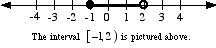index: click on a letter A B C D E F G H I J K L M N O P Q R S T U V W X Y Z A to Z index index: subject areas numbers & symbols sets, logic, proofs geometry algebra trigonometry advanced algebra & pre-calculus calculus advanced topics probability & statistics real world applications multimedia entrieswww.mathwords.com about mathwords website feedback

 Interval Notation A notation for representing an interval as a pair of numbers. The numbers are the endpoints of the interval. Parentheses and/or brackets are used to show whether the endpoints are excluded or included. For example, [3, 8) is the interval of real numbers between 3 and 8, including 3 and excluding 8.Note: Many authors use reversed brackets instead of parentheses. For example, ]5,7[ refers to the interval from 5 to 7, exclusive. The interval in the example below would be written [–1, 2[. See also